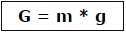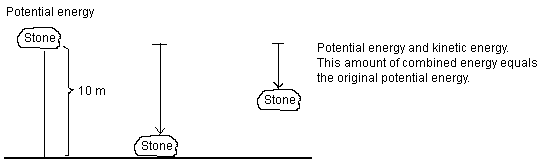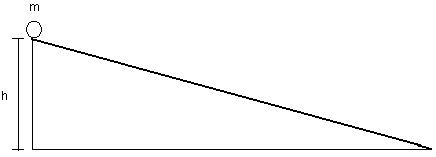## Energy and Work

1. What is mass and what is weight?
2. Give examples of where there is potential energy and where there is kinetic energy?
3. What affects the potential energy and the kinetic energy?
4. What is an inclined plane and what can it be used for considering the above question?
5. Solve simple calculations about work and energy
6. Questions

What is mass and what is weight?

Mass:
The mass is the substance of an object, i.e. how many kilos or grams the object contains. The mass of an object does not change if it moves out into space. The substance of the object is the same.

Weight:
The weight of an object depends on the attractive force towards the center of the Earth. Or, if placed on another planet – the attraction force towards the center of that planet. This attractive force is called gravity.

On Earth the gravitational force is 10 N for each kilo of the object. An object with a mass of one kilogram has the weight 10 N. Similarly; an object with the mass 2 kg has a weight of 20 N.

If however the object is moved to the Sun, the object will have a bigger weight. This is because the Sun has a greater attractive force on the object. This makes an object with the mass 1 kilo having the weight 274 N.

The moon is smaller thus having a lesser attractive force on the object. An object with a mass 1 kilo has a weight of 1.62 N on the moon.

The gravitational force on a planet has to do two things. First, it depends on the planet's mass and secondly, the distance of the object to the planet center. The greater the planet mass is, the greater the gravitational force (attractive force). Further, the closer the object is to the planet center, the greater the gravitational force (attractive force).

Consider an astronaut jumping ahead on the moon. Each jump takes the astronaut higher above the lunar surface compared to if doing the same jump on the Earth's surface.

Weight = Mass (kg) * g-force acceleration (10 m/s2 on earth)G = weight (N)
m = mass (kg)
g = g-force acceleration (10 m/s2 on earth)

Eg. The weight of a kilogram on Earth: G = m * g = 1 kg * 10 m/s2 = 10 N

Give examples of where there is potential energy and where there is kinetic energy?Figure 1: Potential energy converted to kinetic energy

Look at the picture above. A stone is lifted 10 meters above the earth’s surface. The stone has a certain amount of potential energy. This is because the stone can later on drop towards the ground. If the stone falls its potential energy is released.

When the stone is falling towards the ground, the stone’s potential energy is converting gradually into kinetic energy.

At any time during the fall, we can freeze the fall so that the stone is hanging in the air. If we analyze the situation, we can see that the distance the stone has fallen has been converted into kinetic energy. The distance the stone has left to fall can also be converted into kinetic energy, but until then, the remaining energy is potential energy.

Remember that no energy is lost - it can only be converted. On impact all kinetic energy is converted into frictional heat.

What affects the potential energy and the kinetic energy?

Potential Energy:
If an object can fall in a gravitational field, the object has potential energy. Potential energy is relative, meaning that if we lift a spoon and place it on a table, we can say that the spoon has a certain potential energy compared to the floor. If an elevator shaft is right next door, we can say that the spoon has a greater potential energy towards the ground floor of the building. How much energy it is, depends on how long the object can fall, or how high it is lifted.

If the object has a great mass, this will also increase the potential energy as the object is lifted.

Kinetic Energy:
When an object having a certain speed, we say that the object has a certain kinetic energy. The object may be a car traveling on a highway or spoon falling to the ground.

What is an inclined plane and what can it be used for considering the above question?Figure 2: An inclined plane

The ball is at a certain height (h). The ball has a certain mass (m). The height is given in meters and the mass in kilograms. The inclined plane is on the earth where the g-force acceleration (g) is 10 m/s2.

If the ball is released and rolling down the inclined plane the potential energy is gradually more and more converted into kinetic energy. When the ball has rolled all the way down to the ground floor all the potential energy is converted into kinetic energy.

Solve simple calculations about work and energy

Potential Energy:
How big the potential energy is depends on the object’s mass (m), g-force acceleration (g) and how high it is located.

The formula to calculate the potential energy:W = energy (unit: Joule (J))
m = mass (unit: kilograms (kg))
g = g-force acceleration (10 m/s2 on earth)
h = height (unit: meter (m))

Eg.
A stone of 10 kg is lifted 10 m up in the air. What is the stone's potential energy?

m = 10 kg
g = 10 m/s2
h = 10 m
W = ?

==> Wp = m * g * h = 10 kg * 10 m/s2 * 10 m = 1000 J

Answer: The rock's potential energy is 1000 J

Mechanical Work
Mechanical work is done when a constant force is used making an object move a certain distance. If an object is dragged on the ground a friction needs to be continuously overcome. There is required a force to move the object. Mechanical Work is done.

Formula for Mechanical Work:W = energy (unit: joule (J) or Newton meters (Nm))
F = force (unit: Newton (N))
d = distance (unit: meter (m))Figure 3: The mechanical work in this example is 2000 Nm.
A frictional force of 200 N is overcome.

W = F * d = 200 N * 10 m = 2000 Nm

Questions:

1. On which planet would you get the best high jump results - on the Moon or Jupiter?
2. Which of the following planets has the greatest gravitational pull (attractive force) - "Jupiter or Saturn" – why – look in a collection of tables and look for planet gravitations?
3. Explain to a friend what mass is?
4. Explain to a friend what weight is?
5. What is the difference between mass and weight?
6. What is the unit of force?
7. What is the unit of weight?
8. What is the unit of mass?
9. What is g-force acceleration?
10. What is the g-force acceleration on earth and the moon?
11. How do you calculate the weight if you have the mass of an object?
12. What is the weight of each respective mass on earth?
1 kg =                     N
2 kg =                     N
200 grams =                   N
2 t =                        N
0.5 kg =                  N
3 hg =                     N
13. Tell us about your potential energy when you are in school?
14. How do you calculate the potential energy?
15. What determines how big the potential energy is?
16. What is kinetic energy?
17. Describe how potential energy can become kinetic energy?
18. Describe the role of an inclined plane when it comes to describing potential energy and kinetic energy?
19. An object is raised 3 m up in the air. The object has a mass of 3 kg. How big is the potential energy?
20. An object is lifted 5 m up in the air. The object has a mass of 300 kg. How big is the potential energy?
21. An object is lifted 100 m up to the air. The object has a mass of 0.5 kg. How big is the potential energy?
22. An object is raised 3 m up in the air. The object has a weight of 3 N. What is the potential energy?
23. An object is lifted 100 m up in the air. The object has a weight of 1 N. What is the potential energy?
24. An object is dragged 20 m on the ground with the force 50 N. How much mechanical work is done?
25. Felix Mashed potatoes contain 1450 kJ per 100 grams dry matter. How much mashed potatoes do you need to eat in order to drag an object 20 m with the force 20 N? (Then of course, it is needed energy to carry the body load and so on during the trip, but we will not take that into account here).
26. How far can you drag the object in the above question, if you eat 100 grams Felix mashed potatoes?

• NGU Science
• JAN 1, 2012
• FEB 21, 2012
• Admin
##### The course is compiled by Admin

These basic courses are compiled by Lars Helge Swahn. They are designed for 15-16 year old students in order to reach basic skills in science. The courses do also provide an easier way to understand the NGU (AIC) gnostic teachings.

#### Extra information

Copywrite NGU, Northern Pontifical Academy 2019 (A.I.C.)